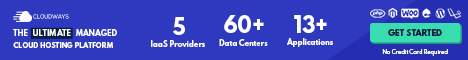# Economic Models

Economists rely on economic theories or models (the words theory and model are used interchangeably) to analyze real-world issues.

Economic models are simplified versions of reality used to analyze real-world economic situations.

Economists are certainly not alone in relying on models: an engineer may use a computer model of a bridge to help test whether it will withstand high winds, or a biologist may make a physical model of a nucleic acid in order to understand its properties better.

One purpose of economic models is to make economic ideas sufficiently explicit and concrete to be used for decision making by individuals, firms or the government.

For example, the model of demand and supply Opens in new window is a simplified version of how the prices of products are determined by the interactions between buyers and sellers in markets.

Economists use economic models to answer questions. For example, consider the question concerning water scarcity: how do we deal with water scarcity in Australia? Opens in new window

For a complicated question such as this, economists often use several models to examine different aspects of the issue.

For example, an economic model which predicts how the price of water affects the amount used would help in analyzing what price should be charged for water.Modeling the costs of alternative ways of supplying water will also help address the problem. Sometimes economists use an existing model to analyze an issue, but in other cases economists must develop a new model.

To develop a model, economists generally follow these steps:

1. Decide on the assumptions to be used in developing the model.
2. Formulate a testable hypothesis.
3. Use economic data to test the hypothesis.
4. Revise the model if it fails to explain well the economic data.
5. Retain the revised model to help answer similar economic questions in the future.

## The Role of Assumptions in Economic Models

Any model is based on making assumptions because models have to be simplified to be useful. We cannot analyze an economic issue unless we reduce its complexity.

For example, economic models make behavioral assumptions about the motives of consumers and firms.

Economists assume that consumers will buy those goods and services that will maximize their wellbeing or their satisfaction. Similarly, economists assume that firms act to maximize their profits.

These assumptions are simplifications because they do not describe the motives of every consumer and every firm.

How can we know if the assumptions in a model are too simplified or too limiting?